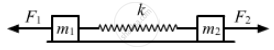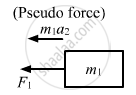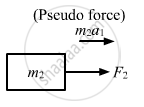Department of Pre-University Education, KarnatakaPUC Karnataka Science Class 11
Advertisement Remove all ads

# Consider the Situation of the Previous Problem. Suppose the Block of Mass M1 is Pulled by a Constant Force F1 and the Other Block is Pulled by a Constant - Physics

Sum

Consider the situation of the previous problem. Suppose the block of mass m1 is pulled by a constant force F1 and the other block is pulled by a constant force F2. Find the maximum elongation that the spring will suffer.

Advertisement Remove all ads

#### Solution

Given:
Force on block of mass, m1 = F
Force on block of mass, m2 =​ F2Let the acceleration produced in mass m1 be a1.
$a_1 = \frac{F_1 - F_2}{m_1 + m_2}$
Let the acceleration of mass m2 be a2.
$a_2 = \frac{F_2 - F_1}{m_1 + m_2}$
Due to the force F2, the mass m1 experiences a pseudo force​.$\therefore \text{ Net force on m}_1 = F_1 + m_1 a_2$

$F' = F_1 + m_1 \times \frac{( F_2 - F_1 )}{m_1 + m_2}$

$= \frac{m_1 F_1 + m_2 F_1 + m_1 F_2 - m_1 F_1}{m_1 + m_2}$

$= \frac{m_2 F_1 + m_1 F_2}{m_1 + m_2}$
Similarly, mass m2 experiences a pseudo force due to force F1$\therefore \text{ Net force on m}_2 = F_2 + m_2 a_1$

$F " = F_2 + m_2 \times \frac{( F_1 - F_2 )}{m_1 + m_2}$

$= \frac{m_1 F_2 + m_2 F_2 + m_2 F_1 - m_2 F_2}{m_1 + m_2}$

$= \frac{m_1 F_2 + m_2 F_1}{m_1 + m_2}$
Let m1 be displaced by a distance x1 and m2 be displaced by a distance ​x2.

Therefore, the maximum elongation of the spring = x1 + x2

Work done by the blocks = Energy stored in the spring

$\Rightarrow \frac{m_2 F_1 + m_1 F_2}{m_1 + m_2} \times x_1 \times \frac{m_2 F_1 + m_1 F_2}{m_1 + m_2} \times x_2 = \left( \frac{1}{2} \right)k( x_1 + x_2 )^2$

$\Rightarrow x_1 + x_2 = \frac{2}{k}\left( \frac{m_1 F_2 + m_2 F_1}{m_1 + m_2} \right)$

Is there an error in this question or solution?
Advertisement Remove all ads

#### APPEARS IN

HC Verma Class 11, 12 Concepts of Physics 1
Chapter 9 Centre of Mass, Linear Momentum, Collision
Q 52 | Page 163
Advertisement Remove all ads
Advertisement Remove all ads
Share
Notifications

View all notifications

Forgot password?